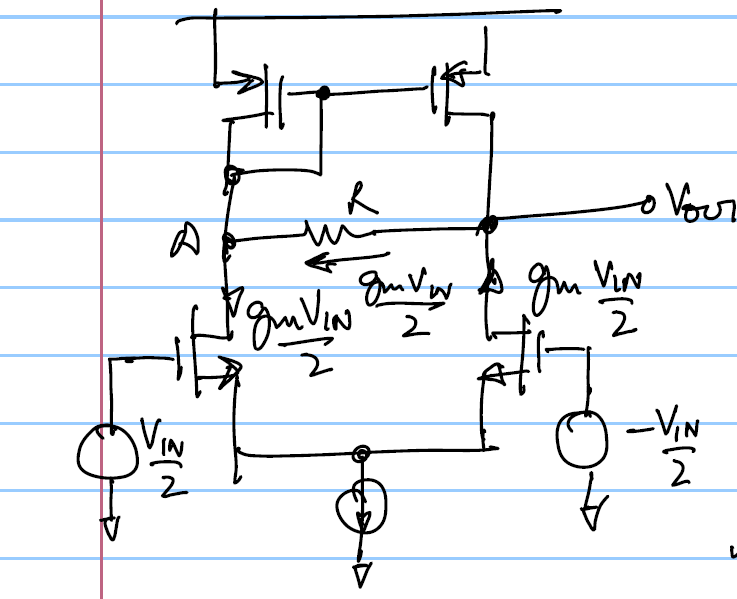# LDO Gain limiting resistor placementThe gain limiting resistor is typically needed to be placed on the high gain stage of the LDO usually an OTA to limit the gain of that stage and also to make the output of that stage the non dominant pole compared to the output pole. Usually in LDO's it is desired to have the LDO output as the dominant pole so that a large cap can be placed there. So all the internal poles have to be pushed out. Adding this gain limiting resistor pushes out the most dominant pole out.

Here 2 ways are shown below to do that on a simple differential amplifier:

# Method 1Here when \$V_{IN}=0\$ voltage across \$R=0\$. When there is some \$V_{IN}\$ then voltage across \$R={g_mV_{IN}R}/2\$
If point A does not change since its a low impedance point we have:
\$\$V_{OUT}={g_mRV_{IN} }/2\$\$

# Method 2In this case:
\$\$V_{OUT}=g_mV_{IN}R\$\$

Thus in this method the resistor required is half the resistor of method 2. But this method will introduce some additional offset since with \$V_{IN}=0\$ some mismatch (from the bias current mismatch and PMOS mismatch) will flow through the resistor causing an input offset on the diffamp.